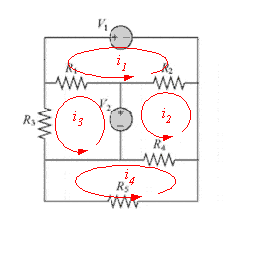# Kirchhoff's Loop Rule Question

lee_sarah76

## Homework Statement

So, I understand that I'm supposed to write the problem however, given the nature of current diagrams I think this is a bit hard. I am given the voltages and resistances and I have to solve for the current through each resistor. Here is a link to the problem which I found online if you wouldn't mind: http://cs.slu.edu/~fritts/csci145/assignments/hwk3.pdf

It is number 6 on that website.

V1 = 12V, V2 = 24V, R1 = 20 ohms, R2 = 12, R3 = 8 ohms, R4 = 6 ohms, R5 = 10 ohms

## Homework Equations

Kirchhoff's Loop Rule states that the sum of the voltages in a closed loop equals 0.

## The Attempt at a Solution

After using Kirchhoff's Loop Rule 5 times I ended up with these set of equations:

V1 - R1(I1 - I3) - R2(I1 - I2) = 0
V2 - R1(I3 - I1) - R3(I3) = 0
-V2 - R2(I2 - I1) - R4(I2 - I4) = 0
0 - R5(I4) - R4(I4 - I2) = 0
V1 - R3(I5 +I3) - R5(I5) =0

However, when using a matrix to solve for I1 through I5, I get: 1.26667, -0.55873, 1.7619, -0.20952, -0.11640.

(Note: I used MATLAB, as this is for a programming class, and my matrices were:
A = [-(R1 + R2), R2, R1, 0, 0; R2, -(R2+R4), 0, R4, 0; R1, 0, -(R1 +R3), 0, 0; 0, R4, 0, -(R5 + R4), 0; 0, 0, -R3, 0, -(R3 + R5)]

B = [-V1; V2; -V2; 0; -V1]

and x = A\B

Obviously, this is not the answer according to the website that the problem statement was on. I believe I am doing something wrong with Kirchhoff's Rule, hence I would be very grateful if someone could help?

## Answers and Replies

Homework Helper
Gold Member
Where and in what direction are your currents defined to be?

If the current In is the current through resistor "n" then this

V1 - r1(i1 - i3) - r2(i1 - i2) = 0

appears wrong to me.

I would expect something more like this for the top loop...

V1 - I1R1 - I2R2= 0

First step is always to mark the currents and their assumed direction. If you have done that post the diagram as it forms part of your working.

Mentor
Hi lee_sarah76. Welcome to Physics Forums.

A couple things. First, there are only four independent loops in the circuit for which you need write an equation. You only need as many loops as required so that at least one current passes through each component. So your fifth loop covers ground already tread by the four previous ones.

While adding the fifth loop doesn't appear to have affected MATLAB's ability to solve the system, it's more work for you, and strictly speaking it actually makes your equations wrong since you didn't account for I5 passing through components in the other equations.

Okay, that said, the results that MATLAB returned are fine! But they are MESH currents. To find the actual current through a given component you must sum the mesh currents that are running through it.

Here's your circuit for easy local reference:#### Attachments

Mentor
@CWatters: I guessed from the layout of the equations that lee_sarah76 was employing mesh analysis. If I'm wrong about that, I am happy to be corrected.

Homework Helper
Gold Member
Yes that makes more sense.

lee_sarah76
Hi! Thank you both CWatters and gneill for answering. @CWatters: As gneill stated, I am attempting to employ mesh analysis, and the direction of the current is the same as gneill posted (Thank you for that!).

@gneill Thank you so much! I overlooked the fact that they were mesh currents when I got the answer and was simply puzzled. However, if you wouldn't mind, I am a little curious about the underlying physics and MATLAB software. You stated that my equations were wrong because I didn't account for I5 components going into the other equations, yet how did I get the right answer for the current through each mesh despite this? Was it simply pure luck? Just curious about this, as I see my mistake from before now!

Mentor
Hi! Thank you both CWatters and gneill for answering. @CWatters: As gneill stated, I am attempting to employ mesh analysis, and the direction of the current is the same as gneill posted (Thank you for that!).

@gneill Thank you so much! I overlooked the fact that they were mesh currents when I got the answer and was simply puzzled. However, if you wouldn't mind, I am a little curious about the underlying physics and MATLAB software. You stated that my equations were wrong because I didn't account for I5 components going into the other equations, yet how did I get the right answer for the current through each mesh despite this? Was it simply pure luck? Just curious about this, as I see my mistake from before now!
It wasn't pure luck. When you tacked on the fifth equation it didn't affect any of the other equations; they still defined the operation of the circuit as given because you didn't include the current I5 in any of their definitions. In essence, the equation for the I5 loop constituted a separate circuit problem which was solved at the same time, in parallel with the problem you were trying to solve. Matrix methods can be very nifty that way. Two problems solved with one "run" of the program.